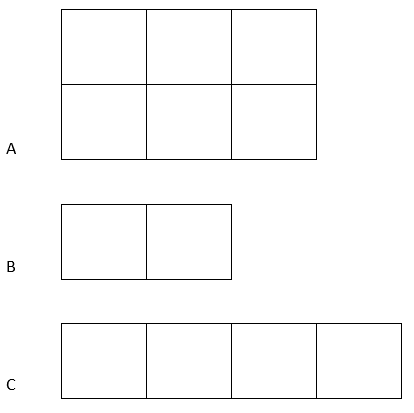Start Now with 100 Free Questions

# HSPT Quantitative Skills Practice Questions

The HSPT Quantitative Skills subtest measures the student’s ability to solve reasoning problems that involve various quantities. It measures a student’s understanding of the four basic math operations, geometric figures, and the relationships between numbers.  The test includes questions covering Number Series, Geometric Comparisons, Non-Geometric Comparisons, and Number Manipulation.

• Number Series – continuing or completing a series or pattern of numbers and/or letters and determining what comes next
• Geometric Comparisons – comparing areas, angles, or other geometric features
• Non-geometric Comparisons – comparing the values of numerical expressions
• Number Manipulation – combining and simplifying numbers by applying the basic operations

Below you will find sample questions that are representative of Quantitative Skills questions your child will see on the test but are not taken directly from the actual HSPT that is being administered this year.

1. Look at this series: 3, 7, 15, 31, 63. What number comes next in the series?

A. 97
B. 98
C. 127
D. 128

2. Examine figures A, B, and C and find the best answer.A. A > B + C
B. C < 2B
C. A + B < C
D. A – B = C

3. Examine (a), (b), and (c) and find the best answer.

(a) 4(3 – 1)
(b) 4 x 3 – 1
(c) 3(-1 x 4)

A. (b) < (a) + (c)
B. (b) = (a)
C. (a) + (b) > (c)
D. (b) + (c) > (a)

4. What number is 10 more than 22% of 100?

A. 40
B. 32
C. 122
D. 22

Are you ready to start preparing your child for the HSPT? Are you interested in more HSPT practice? We have over 1,700 HSPT practice questions, plus expert tutors, engaging test prep, and more! Try out our 100 Free Questions today!

100 Free Practice Questions